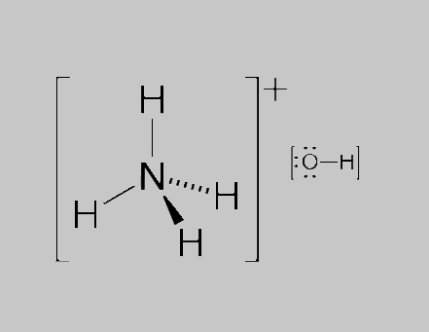# NH4OH - Ammonium Hydroxide

## What is NH4OH (Ammonium Hydroxide)?

NH4OH is the chemical formula of a solution of ammonia in water. This solution is known by various names such as ammonia water, ammonium hydroxide, ammonia liquor, and aqueous ammonia. NH4OH is often denoted by the symbol ‘NH3 (aq)’.Ammonium Hydroxide (NH4OH)

The general structure of an NH4OH molecule is illustrated above.

## Basicity of NH4OH

When ammonia is dissolved in water, the water molecules donate a proton to the NH3 molecule. This leads to the formation of an ammonium cation (whose chemical formula is NH4+) and a hydroxide ion (OH). This can be represented by the following equilibrium reaction.

NH3 + H2O ⇌ NH4+ + OH

A solution of ammonia in water with a concentration of 1M displays a 0.42% conversion of ammonia into ammonium cation.

## Saturated Solutions of NH4OH

The solubility of ammonia decreases with the increase in temperature of the water solvent. It can be observed that this behaviour of ammonia is quite similar to that of other gases. The solution of ammonia in water exhibits a decrease in its density with the increase in the concentration of dissolved ammonia.

The density of a saturated ammonium hydroxide solution at 288.75 K is equal to 0.88 grams per mole. This saturated solution of ammonium hydroxide would contain approximately 35.6% ammonia by mass which corresponds to 308 grams of ammonia per litre of saturated ammonium hydroxide solution.

## Properties of Ammonium Hydroxide (NH4OH)

An NH4OH solution has the following properties in its standard state:

• NH4OH has a molar mass of 35.04 grams per mole.
• It has a colourless appearance in its liquid state.
• Ammonium hydroxide solutions have a highly pungent, “fishy” odour.
• It has a density of 0.91 grams per cubic centimetre for a 25% w/w solution. A 35% w/w solution would have a density of 0.88 grams per cubic centimetre.
• Ammonium hydroxide solution with a 25% mass fraction has a melting point of 215.7 K and a boiling point of 310.8 K
• Its standard enthalpy of formation is -80 Kilojoules per mole.

## Uses of Ammonium Hydroxide (NH4OH)

Ammonia water has many applications in industry as well as in laboratories. Five such uses of ammonia solutions are listed below.

• Ammonium hydroxide solution is an important tool in the manufacturing process of chemical fertilizers. It is used as a solution or as salt in these fertilizers.
• Ammonium hydroxide is also used in the production of organic and inorganic chemicals containing nitrogen. It is the base chemical in the manufacture of nitric acid.
• A 1-3% solution of NH4OH is used in cleaning agents such as window cleaning liquids. A solution of ammonia in water (scented or plain) is also sold directly as a cleaning agent.
• Ammonia can be used in the production of chloramine which is a good disinfectant. It remains active in still water for longer durations than chlorine.
• Any wood that contains tannic acid can be sealed in a container with ammonium hydroxide solution to give a dark stained look to the wood. Thus, the ammonium hydroxide solution is used in furniture darkening.